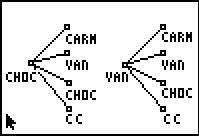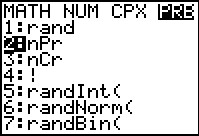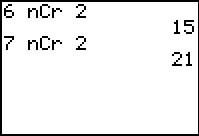# Activities

••• ##### Subject Area

• Math: Statistics: Probability and Random Variables

• ##### Author9-12

40 Minutes

• ##### Device
• TI-83 Plus Family
• TI-84 Plus
• TI-84 Plus Silver Edition
•TI-84 Plus C Silver Edition
•TI-84 Plus CE

## Too Many Choices!#### Activity Overview

Students investigate the fundamental counting principle, permutations, and combinations.

#### Key Steps

•Students will explore the fundamental counting principle and discover its formula. They will use diagrams to help them determine the number of different combinations of cake and frosting. After a discussion of the formula, students will use it to solve several problems.

•Then, students will use the fundamental counting principle to discover the general formula for permutations. In this investigation students will determine the number of arrows that connect 2 points for a given number of possible points. After a discussion of the formula, students will use it to solve several problems.

•Students will use the general formula for permutations to discover the formula for combinations. They will determine the possible combinations for a committee of 3 people selecting from 6 people. Then students will continue their investigation of the arrows by determining the number of edges, where the order of the arrows does not matter. After a discussion of the formula, students will use it to solve several problems.